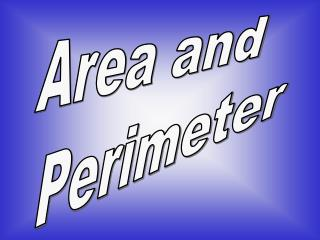DownloadDownload PresentationArea and Perimeter

# Area and Perimeter

Download Presentation## Area and Perimeter

- - - - - - - - - - - - - - - - - - - - - - - - - - - E N D - - - - - - - - - - - - - - - - - - - - - - - - - - -
##### Presentation Transcript

1. Area and Perimeter

2. Area Area is the amount of surface space that a flat object has. Area is reported in the amount of square units.

3. When you measure the amount of carpet to cover the floor of a room, you measure it in square units. ? Would the area of your bedroom or the area of your house be greater? ? You’re right! The area of your house is greater than the area of your bedroom.

4. Lets find the area of this surface if each square is equal to one foot. Count the number of squares. 1 2 Area = 15 square feet 3 4 5 6 7 8 9 10 11 12 13 14 15

5. Try this one! Count the number of green squares to determine the area of this surface. What is the area? 1 2 3 4 5 6 7 8 9 The area is equal to 9 square units.

6. Perimeter Perimeter is the distance around the outside edge of a flat object. Perimeter is reported as a total number of linear units.

7. When you measure the amount of wallpaper border to go around a room, you measure it in lengths. ? Would the perimeter of your bedroom or the perimeter of your house be greater? ? You’re right! The perimeter of your house is greater than the perimeter of your bedroom.

8. Let’s find the perimeter of this surface if each square is equal to one foot. Count the number of sides. Perimeter = 24 feet

9. Try this one! Count the number of sides to determine the perimeter of this flat object. The perimeter is equal to 12.

10. Family A Family B Let’s do these problems together. Two neighbors build swimming pools. This is what the pools look like. Which family has the pool with the bigger swimming area?

11. Family A Family B The area of Family A’s pool is? 8 square units. The area of Family B’s pool is? 7 square units Therefore, Family A has the pool with the bigger swimming area.

12. Family A Family B Now look at those same two pools. Which family has more side panels of the pool to clean? The perimeter of Family A’s pool is 12 units long. The perimeter of Family B’s pool is 14 units long. Therefore, Family B has more side panels of the pool to clean.

13. The End